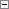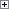This simple data type stores whole numbers with values that range from -2,147,483,647 to 2,147,483,647.Remarks

In addition to representing whole numbers in this range, you can use integers to represent Boolean values. For Boolean values, -1 represents true and 0 represents false.

If you assign -2,147,483,648 directly to an Integer variable, then you get an error when you try to compile the code. However, you can indirectly assign -2,147,483,648 to an Integer variable by using the following code.Copy Code
IntegerVar := -2147483647;
IntegerVar := IntegerVar - 1;

If you try to indirectly assign a value that is smaller than -2,147,483,648 or larger than 2,147,483,647, then you get a run-time error.Example

The following are examples of integer values.Copy Code
546
-3425

The following example is a decimal and not an integer.Copy Code
342.45